# AP Physics C: Mechanics : Understanding Newton's Laws

## Example Questions

### Example Question #1 : Newton's Laws And Force Diagrams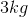paint bucket hangs from the right end of a meter stick, oriented horizontally. The left end of the meter stick rests on a fulcrum so that it may rotate about that point. A rope is to be attached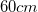from the left end, so that the system does not rotate. What is the minimum force this rope must support, assuming the meter stick is massless?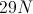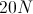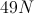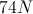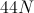Explanation:

Relevant equations: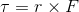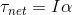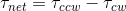Determine the clockwise torque caused by the bucket.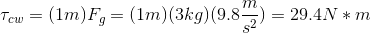Write an expression for the counterclockwise torque caused by the rope.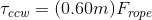Combine the torque of the rope and the torque of the bucket into the net torque equation.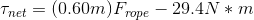Since the system has no angular acceleration, net torque must be zero, allowing us to solve for the force of the rope.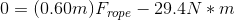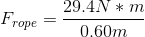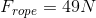### Example Question #1 : Newton's Laws And Force Diagrams

An object at rest will remain at rest unless acted on by a(n) __________.

internal force

positive force

external force

negative force